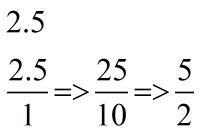Request a Tool

Decimal To Fraction Calculator

Decimal To Fraction Calculator is use to convert decimal value to fraction form.

Fraction
0

Step1

Write down the decimal divided by 1

Step2

Multiply both top and bottom by 10 for every number after the decimal point

Step3

Simplify (or reduce) the fraction.

ExampleDefination / Uses

To convert a decimal to a fraction, position the decimal number above its place value. For example, because the six in 0.6 is in the tenths position, we multiply 6 by 10 to get 6/10, which is an equal fraction.

The method of converting a decimal to a fraction is simple. Simply multiply the value by 10 to the power of whichever many times is required to leave no significant figures after the decimal point (N). Then write that amount as the numerator of the fraction. Write 10 to the power of N as the denominator, where N is the number of significant digits after the decimal point. As a result, the final number is the original number multiplied by 10N times 10N. After that, this fraction can be simplified by expressing it as a mixed fraction.